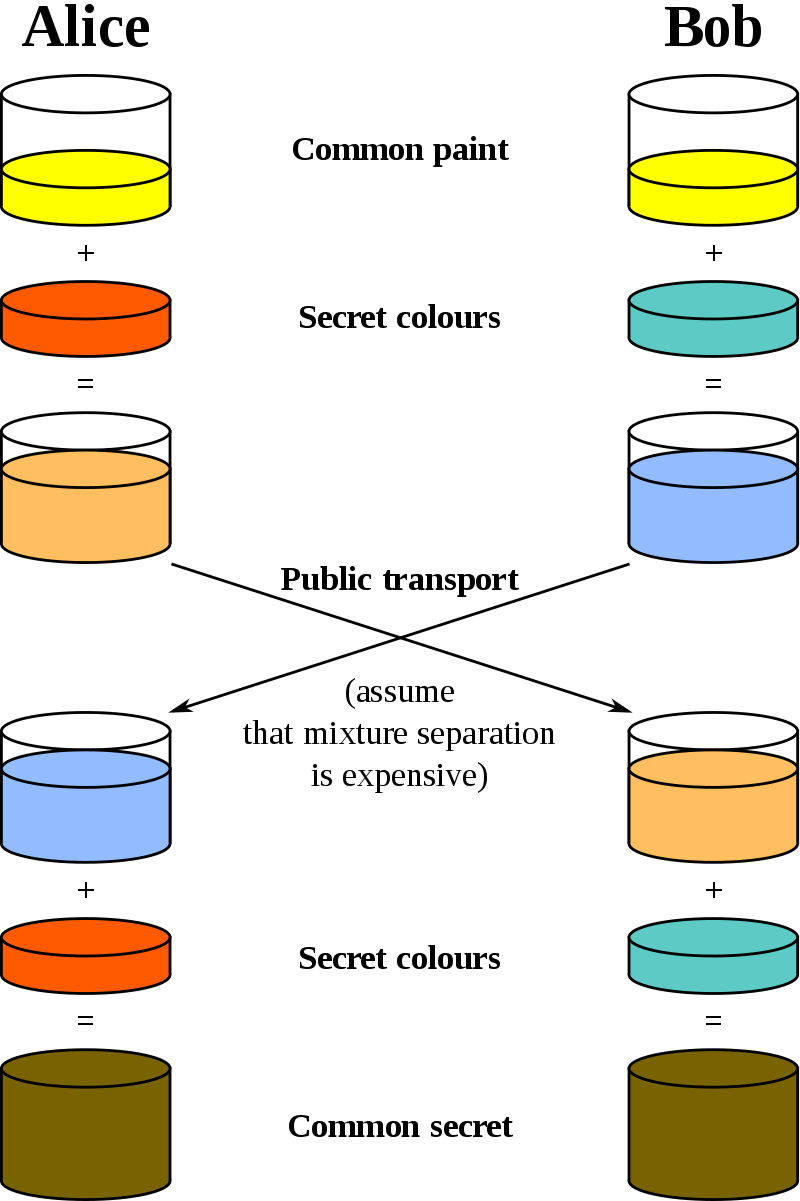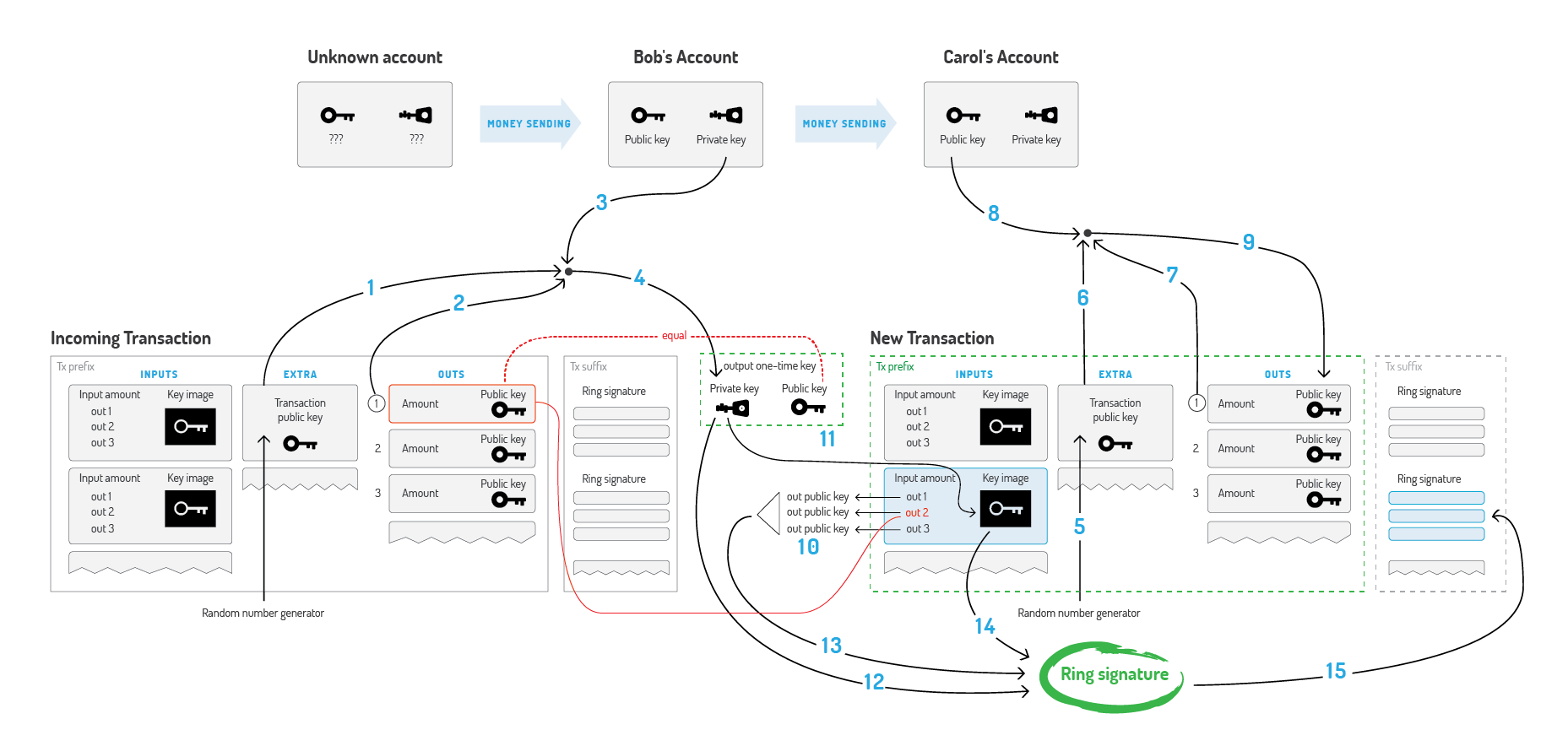# 匿名链中的密码学

$r\xrightarrow{\times G} R$，但 $R\stackrel{\div G}\nrightarrow r$

Alice：我们先选出一个大数G，这个G所有人都可以知道。然后我在心里面想一个大数a，我把a乘以G的结果，假设是A告诉Bob，当然A是多少也被大家听到了。

Bob：好的，现在我也在心里想一个大数b，并且把b乘以G的结果B告诉Alice。

Alice：明白了，那么最终敲定的密钥就是a乘以B的结果，这个用计算机很快就能算出来。

Bob：算出来的结果不用告诉我，因为我拿b乘以A得到的结果是一样的。

$(g^a\;mod\;p)^{b}\;mod\;p=(g^b\;mod\;p)^{a}\;mod\;p$，其中p是素数，g是p的本原根。1. Alice将m编码后计算：M=m+aB。
2. Bob拿到M计算：m=M-bA，完成。

1. Bob向Alice公开了他的标准地址（比如说扫一扫），这个地址不是直接用于收款的，而是存储了两个公钥（$B_1、B_2$），任何人都能获得，他自己则保留对应的两个私钥（$b_1、b_2$）。$B_1=b_1G,B_2=b_2G$,这里G是椭圆曲线上选取的基点，全网统一，同样的算法基点都是一样的.
门罗标准地址格式：网络编码(1 byte)+public spend key(32 byte)+public view key(32 byte)+校验和(4 byte)
2. Alice随机生成了一个整数r作为另一个私钥，套用公式$P=H_s(rB_1)G+B_2$得[一次性]公钥P，其中$H_s$是一个哈希函数。P就是Bob的一次性收款地址（stealth address）。
思考$H_s$的作用？
3. Alice使用P作为转账的目标地址，同时将$R=rG$打包进此次交易。注意她可以使用相同的r进行不同目标地址的转账。
4. Alice将交易发送至链，因此P、R也一同被公开到链上。
P中的$H_s$不但规范了P的数据结构（长度），同时也避免第三方将P与Bob关联起来。试想，若无$H_s$，那么$P=rB_1G+B_2=RB_1+B_2$，第三方很容易通过试算得到实际的收款方。
5. Bob扫描区块链上[自上次扫描以来的]最近交易，计算$P'=H_s(b_1R)G+B_2$。如果P’=P，则说明这笔转账是给Bob的，因为$b_1R=b_1rG=rb_1G=rB_1$。
6. Bob要花这笔钱（转账给别人），则首先要计算出与P对应的私钥x，即xG=P，由$P=P'=H_s(b_1R)G+B_2$可得：$x=H_s(b_1R)+b_2$。这个私钥连给钱的Alice也无法推出（因为有$b_2$项），日后Bob就可以使用这个私钥来花这笔钱了。

1. 随机选取n-1个公钥，连同自己的公钥$P_s$组成n个公钥集合$\{P_1,P_2,\cdots ,P_s\cdots ,P_n\}$；
2. 随机选取n-1个值$\{x_1,x_2,\cdots ,x_n\}$，其中$x_s$暂缺，后面会使用计算得到的合适值补上；
这两步和之前的例子一样。
3. 设一未知数$v_1$，再设$Y_1=x_1G+v_1P_1$；
4. 设一未知数$l$，再设$R_1=x_1H_p(P_1)+v_1l$；
注意$l,R_i$存在与否并不影响环签名，而是另有考虑，可看下节Key Image，此节可忽略。
5. 计算$v_2=H_s(m,Y_1,R_1)$，其中$H_p$和$H_s$是两个不同的哈希函数，m是需要签名的数据，根据业务需求来；
6. 同上依次计算$Y_2=x_2G+v_2P_2;R_2=x_2H_p(P_2)+v_2l;v_3=H_s(m,Y_2,R_2);\cdots ;Y_n=x_nG+v_nP_n;R_n=x_nH_p(P_n)+v_nl;v_{n+1}=H_s(m,Y_n,R_n)$；
7. 令$\underline{v_{n+1}=v_1}$，这就是我们要构造的方程，为了使方程成立，关键落在寻找合适的$x_s$及未知数$v_1,l$上；
8. 虽然每一环的$v$由上一环而来，但是由于其首尾相连，我们无法由任一环推出其值，也就是说其值是不定/任意的；那么我们可以先随意设置某环的计算结果，再反推出符合该结果的$v$值。因此任选一个数a，使得$Y_s=aG,R_s=aH_p(P_s)$，$Y_s,R_s$一旦确定，$v_{s+1}=H_s(m,Y_s,R_s)$也跟着确定下来，继而$v_{s+2},v_{s+3},\cdots ,v_n,v_1,\cdots ,v_{s}$都可依次推得；
9. 又$Y_s=x_sG+v_sP_s$，易得$x_s=a-v_ss_s$，其中$s_s$是$P_s$对应的私钥。如此，$\mathbb{X}$被补足，现在只剩$l$未知；
10. 由$R_s=x_sH_p(P_s)+v_sl=aH_p(P_s)$及$a=x_s+v_ss_s$，易得$l=s_sH_p(P_s)$。
11. 证明方将$\mathbb{P}$、$\mathbb{X}$、$v_1$、$l$公开到链上，作为交易的发起者；矿工验证。

Key Image

monero也使用UTXO，毕竟匿名性就决定了交易/余额不能基于账户模型，但与比特币不同，由于环签名的缘故，矿工无法确定一个交易中的实际UTXO是哪个，自然无法将其从数据库中标记删除，从这个角度说，UTXO一旦产生，就永远有效，这样显然不合理。门罗币使用Key Image解决这个问题。简单来说，一个Key Image对应一个UTXO（UTXO被花费的同时产生对应的Key Image），矿工只要查看这次交易产生的Key Image是否存在，即可判断UTXO是否被重复消费。上述的$l$就承担了Key Image的职责。

Alice 在给 Bob发送XMR的时候，其实是将自己的一笔或多笔UTXO变成Bob的一笔UTXO（当然一般还包括矿工费及找零，此处忽略）；

2017年1月10日Monero中正式使用了RingCTs。而在此之前，Monero中假如Alice需要向Bob转12.5个XMR，则需要将自己的UTXO分别发送至三个地址，分别转账10XMR、2XMR和0.5XMR，这是因为Monero中要求转账的XMR格式为A×10BA×10B的格式，环签名中诱骗的UTXO也要相同数额。使用了RingCTs之后，在区块链上隐藏了交易数额，这时候Alice挑选mixins的时候，不用考虑mixins的XMR的余额，提升了交易的隐私性。

Alice需要花费的真实UTXO有几笔，该过程就重复几次。Ring Confidential Transactions

OK，目前为止，我们实现了在一次转账中：

• 隐藏转账方[UTXO[一次性]公钥地址]（环签名）。
• 隐藏了收款方[标准公钥地址]（一次性地址）。
• 防止双花（Key Image）。

1. 首先，输入=输出。这很好理解，Alice支出的钱必须得等于(Bob收到的钱+矿工费+找零)，收支平衡，只要保证货币初始来源合法（如挖矿），那么追根溯源，后续的UTXO必然合法，然而这还不够。
2. 假如Alice构造了一笔交易输入=1BTC，输出=1000BTC+(-999)BTC，不考虑矿工费，其中1000BTC转给自己的另一个地址，-999BTC转给原地址作为找零，后续她可以不管原地址，而使用新地址，如此则凭空多出了巨额财富。要避免这种情况很简单，只要规定(每笔输入输出>0)。

Pedersen commitments

C_{in}=xG+aG\Longrightarrow \left\{\begin{aligned}C_{out-1}=y_1G+b_1G\\C_{out-2}=y_2G+b_2G\end{aligned}\right.。

monero构造上述承诺稍有不同，留待以后讲解。

1. 将金额b表示成二进制形式：$b=b_02^0+b_12^1+\cdots +b_n2^n,\ b_i\in \{0,1\}$；
2. 将私钥y对应拆分为n个随机数之和：$y=y_0+y_1+\cdots +y_n$；
3. 根据加法同态，C可表示为：$C=C_0+C_1+\cdots +C_n$，其中$C_i=y_iG+(b_i2^i)H$；
4. 针对每个私钥$y_i$，取两个公钥$\{C_i,C_i-2^iH\}$，两者必有一个等于$y_iG$，所以真正的密钥对为$<y_i,y_iG>$。将该公钥对进行环签名形成二元环签名，表明构造者持有这两个公钥的其中一个私钥。有多少个私钥，就对应多少个二元环签名；
5. 矿工只需进行两步验证：1、$C_i$之和是否等于C；2、各个环签名是否正确。

https://cryptonote.org/whitepaper.pdf